Verifying Zeroes of a polynomial

Chapter 2 Class 9 Polynomials
Concept wise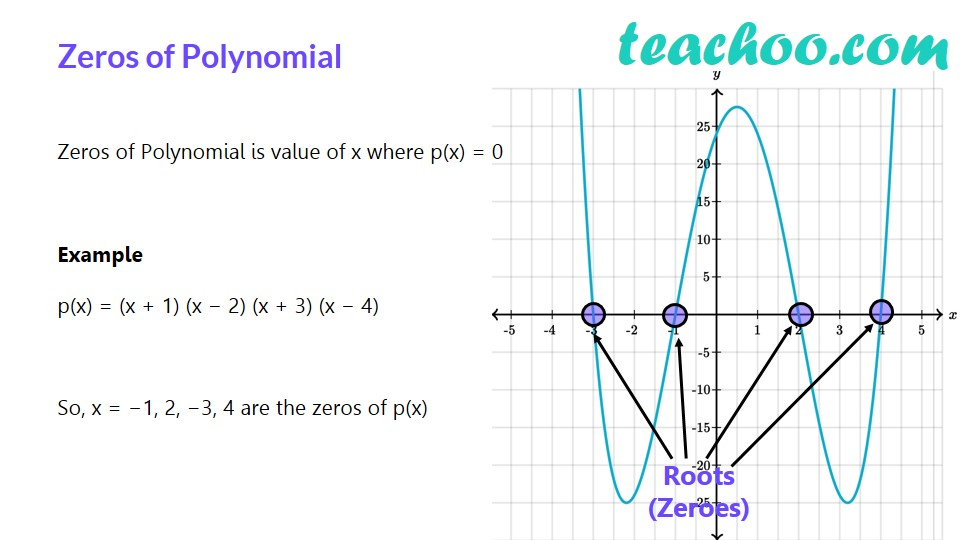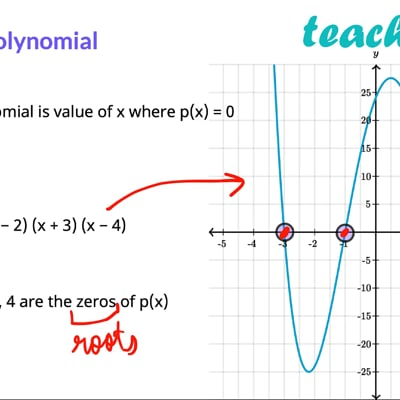This video is only available for Teachoo black users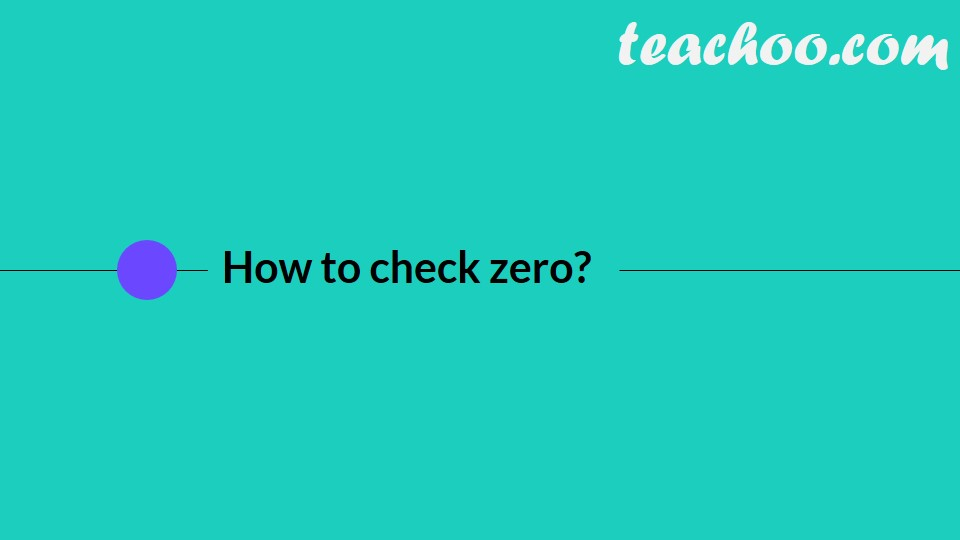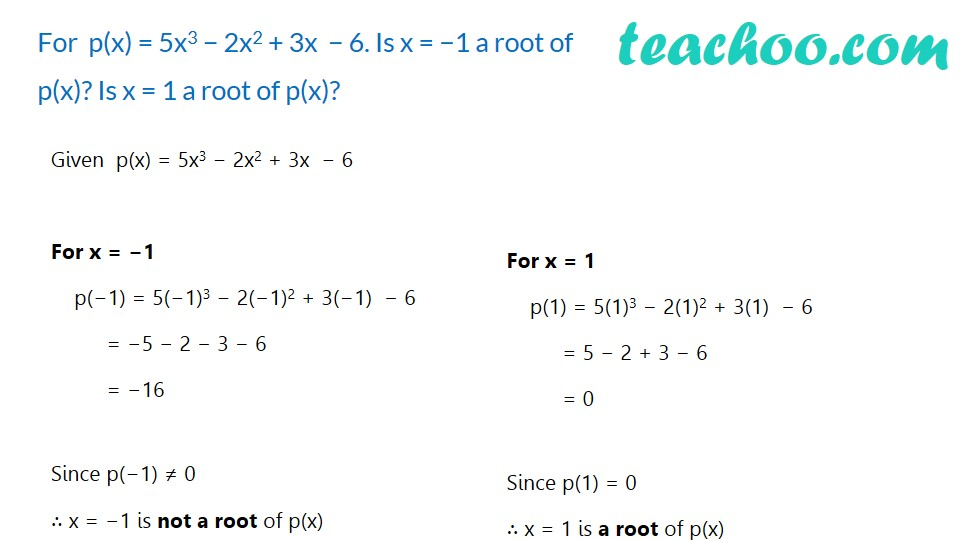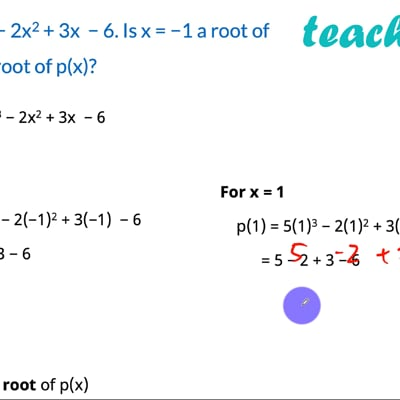This video is only available for Teachoo black users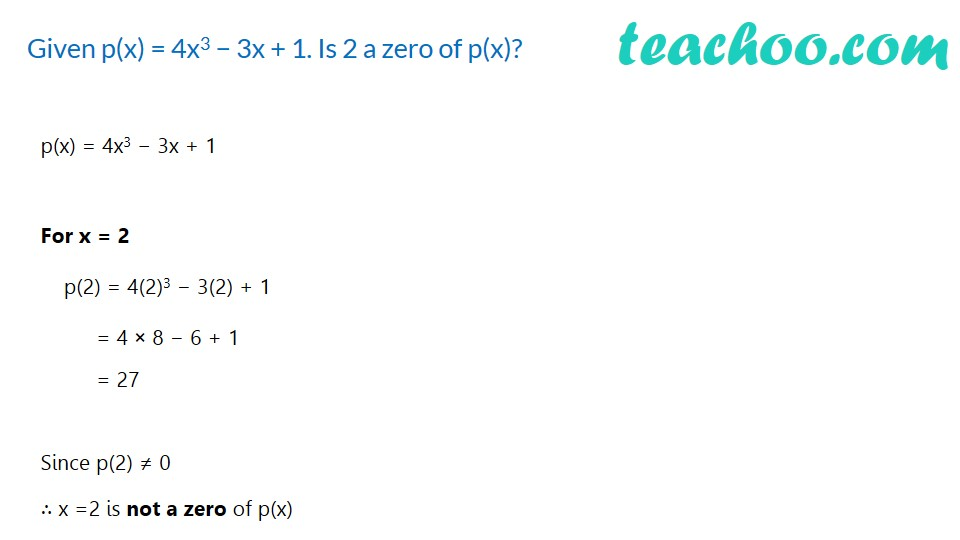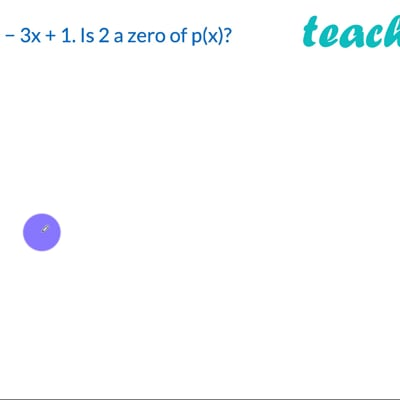This video is only available for Teachoo black users

For polynomial  p(x) ,

If  p(a) = 0

Then x = a is the zero of polynomial

Let us take an example

Let  p(x) = 5x 3 − 2x 2 + 3x  − 6

For x = 1

p(1) = 5(1) 3 − 2(1) 2 + 3(1)  − 6

= 5 − 2 + 3 − 6

= 5 + 3 − 2 − 6

= 0

So p(1) = 0 for x = 1

∴ x = 1 is a zero of p(x)

Next, we will learn how to find zeroes of a polynomial

Solve all your doubts with Teachoo Black (new monthly pack available now!)